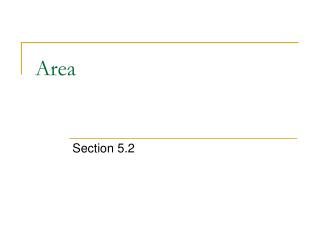DownloadDownload PresentationArea

# Area

Download Presentation## Area

- - - - - - - - - - - - - - - - - - - - - - - - - - - E N D - - - - - - - - - - - - - - - - - - - - - - - - - - -
##### Presentation Transcript

1. Area Section 5.2

2. Overview: Summation Formulas: Rectangular Area: Area under the curve:

3. Summation Formulas: (back)

4. y = f(x) Rectangular Area: Area of a Rectangle = base * height A = height x base A = height · ∆x ∆x A = y · ∆x A = f(x)· ∆x (back)

5. Area under the curve: Approximating Area using Rectangles and Summations RAM : Rectangular Approximation Method LRAM MRAM RRAM Demo: Class Example (RAM): Unlimited / Infinite amount of Rectangles… (back)

6. Class Example: Estimate the area under the curve from [0,2] for y = -x^2+5 using RRAM with five rectangles (back)

7. Infinite Number of Rectangles Add more rectangles (back)